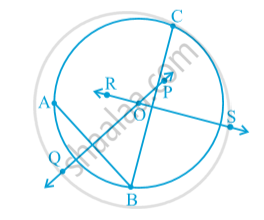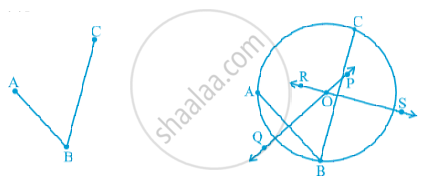# Circles Passing Through One, Two, Three Points

#### notes

We know that through one point say 'P' we can draw many circles.Through two point say 'P' and 'Q' again we can draw many circles.Now take three points 'A' , 'B' and 'C'.
After drawing perpendicular bisector AB on 'PQ' and RS on 'BC', we find that OA = OB = OC.Where O is the point where both the perpendicular bisector meet.
Now a circle can be drawn by taking O as a centre and OA as a radius. This demonstration proves the theorem that  There is one and only one circle passing through three given non-collinear points.
This circle is called the circumcircle of the ∆ ABC. Its centre and radius are called respectively the circumcentre and the circumradius of the triangle.

#### theorem

Theorem :  There is one and only one circle passing through three given non-collinear points.

Proof :Given : We have three non -collinear points A , B and C (As shown e fig.)
To prove : We need to prove that there is ONE and ONLY one circle that passes through all the three points A , B and C.
We join AB and BC . Then we draw a perpendicular bisector of AB say line QP . Also draw a perpendicular bisector of BC say line RS .
Since AB and BC are not parallel , their perpendicular bisector i.e. line QP and line RS will not be parallel. Say line QP and line RS intersect at point O.

Now we know that if we take any point on the perpendicular bisector of AB , that point will be equidistant from both A and B . So, O is on line QP.

Therefore , OA = OB  .....(1)

Similarly , if we take any point on the perpendicular bisector of BC , that point will be equidistance from both B and C. So, o is on line RS.

Therefore, OB = OC  ....(2)

From (1) and (2) , we get
OA =OB =OC

We see that O is such a point that is equidistant from all three points A,B and C. So, we can draw a circle with centre O and radius OA that will passed through B and C (since OA =OB = OC).

Since O is a unique point i.e. the point of intersection of perpendicular bisectors of AB and BC and we saw that OA=OB = OC , we can say that there is ONE and OnLY one circle that passes through all three non - collinear points.
Hence proved.

#### description

• Infinite circles pass through one point.
• Infinite circles pass through two distinct points.
• There is a unique circle passing through three non-collinear points.
• No circle can pass through 3 collinear points.
If you would like to contribute notes or other learning material, please submit them using the button below.

### Shaalaa.com

Theorem : There is one and only one circle passing through three given non-collinear points. [00:21:21]
S
0%